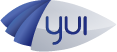#API Docs for: 3.5.1
Show:

# Drawing Class

`Drawing` provides a set of drawing methods used by `Path` and custom shape classes. `Drawing` has the following implementations based on browser capability.

()

## Methods

()

Clears the path.

### `curveTo`

(
• `cp1x`
• `cp1y`
• `cp2x`
• `cp2y`
• `x`
• `y`
)

Draws a bezier curve.

#### Parameters:

• `cp1x` Number

x-coordinate for the first control point.

• `cp1y` Number

y-coordinate for the first control point.

• `cp2x` Number

x-coordinate for the second control point.

• `cp2y` Number

y-coordinate for the second control point.

• `x` Number

x-coordinate for the end point.

• `y` Number

y-coordinate for the end point.

### `drawRect`

(
• `x`
• `y`
• `w`
• `h`
)

Draws a rectangle.

#### Parameters:

• `x` Number

x-coordinate

• `y` Number

y-coordinate

• `w` Number

width

• `h` Number

height

### `drawRoundRect`

(
• `x`
• `y`
• `w`
• `h`
• `ew`
• `eh`
)

Draws a rectangle with rounded corners.

#### Parameters:

• `x` Number

x-coordinate

• `y` Number

y-coordinate

• `w` Number

width

• `h` Number

height

• `ew` Number

width of the ellipse used to draw the rounded corners

• `eh` Number

height of the ellipse used to draw the rounded corners

### `end`

()

Completes a drawing operation.

### `lineTo`

(
• `point1`
• `point2`
)

Draws a line segment using the current line style from the current drawing position to the specified x and y coordinates.

#### Parameters:

• `point1` Number

x-coordinate for the end point.

• `point2` Number

y-coordinate for the end point.

### `moveTo`

(
• `x`
• `y`
)

Moves the current drawing position to specified x and y coordinates.

#### Parameters:

• `x` Number

x-coordinate for the end point.

• `y` Number

y-coordinate for the end point.

### `quadraticCurveTo`

(
• `cpx`
• `cpy`
• `x`
• `y`
)

#### Parameters:

• `cpx` Number

x-coordinate for the control point.

• `cpy` Number

y-coordinate for the control point.

• `x` Number

x-coordinate for the end point.

• `y` Number

y-coordinate for the end point.